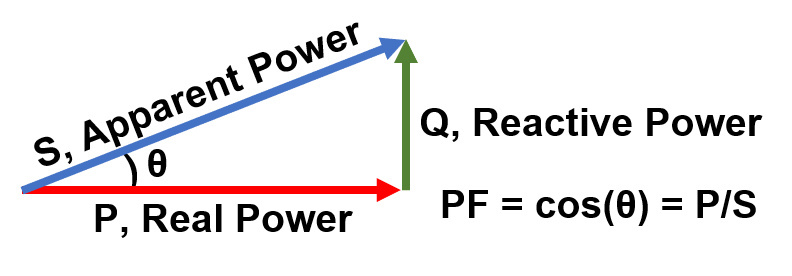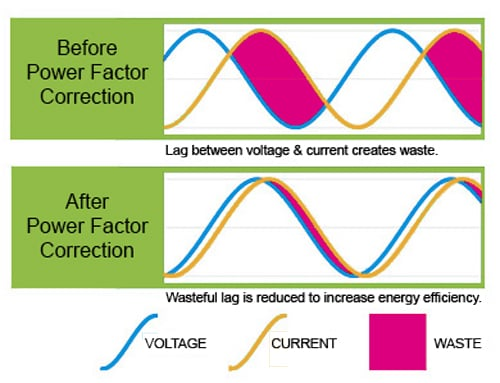# Power Factor Correction

Power factor is defined as the ratio of the real power to the apparent power in a circuit. Essentially, real (or active) power is the amount of power that is actually usable, while reactive power is waste or unusable power. Apparent power is the vector sum of real power and unusable reactive.The phenomenon of real power and reactive power occurs due to the phase difference between voltage and current. It’s an inherent result of inductance and capacitance in any AC circuit. This frequent lag causes results in only some of the power being useful. Correcting the power factor reduces the power that is wasted.

An ideal power factor would be 1.0, as the range is from 0 to 1. Because inductance is always changing with the load, maintaining a perfect power factor of 1.0 is impractical and often impossible. However, Power Factor Correction (PFC) procedures can keep the power factor within an acceptable range. For some applications, correcting the power factor in an AC circuit can be as simple as adding a capacitor in parallel with the electrical load. For others, it can be more complex.For non-residential locations, uncorrected or poorly corrected power factors will usually result in higher electric bills since the utility company has to compensate for reactive power losses in the form of penalties and surcharges.  Several countries also have legislation regarding acceptable minimum power factors.

Uncorrected power factors also raise the risk of harmonic distortion in the current and voltage cycles when non-linear loads are connected to the system. Many electrical components rely on precisely controlled current and voltage to function properly, but harmonics polluting the power line results in voltage fluctuation, which limits the power and speed range of motor drives. In short, harmonic distortion can cause devices to become unstable, overheat, or even fail altogether.

Methods of improving power factor, such as using power supplies with PFC capabilities, are a worthwhile investment.  The advantages of power factor improvement include:

• Greater efficiency reduces electricity costs
• Cleaner power on factory floor
• Cleaner voltage output over a wide range of inputs
• Protection for valuable equipment
• Better machine reliability in difficult conditions
• No extra surcharges from the power company

Power factor is defined as the ratio of the real power to the apparent power in a circuit. Essentially, real (or active) power is the amount of power that is actually usable, while reactive power is waste or unusable power. Apparent power is the vector sum of real power and unusable reactive.The phenomenon of real power and reactive power occurs due to the phase difference between voltage and current. It’s an inherent result of inductance and capacitance in any AC circuit. This frequent lag causes results in only some of the power being useful. Correcting the power factor reduces the power that is wasted.

An ideal power factor would be 1.0, as the range is from 0 to 1. Because inductance is always changing with the load, maintaining a perfect power factor of 1.0 is impractical and often impossible. However, Power Factor Correction (PFC) procedures can keep the power factor within an acceptable range. For some applications, correcting the power factor in an AC circuit can be as simple as adding a capacitor in parallel with the electrical load. For others, it can be more complex.For non-residential locations, uncorrected or poorly corrected power factors will usually result in higher electric bills since the utility company has to compensate for reactive power losses in the form of penalties and surcharges.  Several countries also have legislation regarding acceptable minimum power factors.

Uncorrected power factors also raise the risk of harmonic distortion in the current and voltage cycles when non-linear loads are connected to the system. Many electrical components rely on precisely controlled current and voltage to function properly, but harmonics polluting the power line results in voltage fluctuation, which limits the power and speed range of motor drives. In short, harmonic distortion can cause devices to become unstable, overheat, or even fail altogether.

Methods of improving power factor, such as using power supplies with PFC capabilities, are a worthwhile investment.  The advantages of power factor improvement include:

• Greater efficiency reduces electricity costs
• Cleaner power on factory floor
• Cleaner voltage output over a wide range of inputs
• Protection for valuable equipment
• Better machine reliability in difficult conditions
• No extra surcharges from the power company